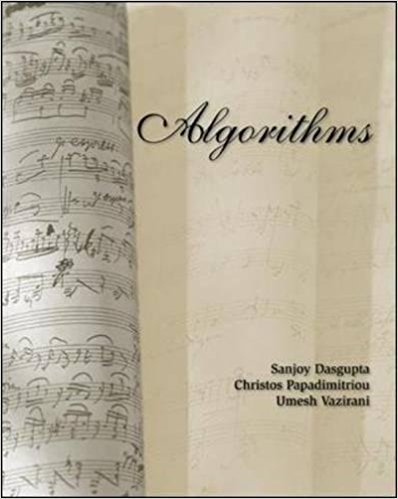×
×

# Solutions for Chapter 1: Algorithms with numbers## Full solutions for Algorithms | 1st Edition

ISBN: 9780073523408Solutions for Chapter 1: Algorithms with numbers

Solutions for Chapter 1
4 5 0 414 Reviews
11
0
##### ISBN: 9780073523408

This expansive textbook survival guide covers the following chapters and their solutions. Since 46 problems in chapter 1: Algorithms with numbers have been answered, more than 17079 students have viewed full step-by-step solutions from this chapter. Chapter 1: Algorithms with numbers includes 46 full step-by-step solutions. Algorithms was written by and is associated to the ISBN: 9780073523408. This textbook survival guide was created for the textbook: Algorithms , edition: 1.

Key Statistics Terms and definitions covered in this textbook
• Analytic study

A study in which a sample from a population is used to make inference to a future population. Stability needs to be assumed. See Enumerative study

• Average

See Arithmetic mean.

• Bias

An effect that systematically distorts a statistical result or estimate, preventing it from representing the true quantity of interest.

• Contingency table.

A tabular arrangement expressing the assignment of members of a data set according to two or more categories or classiication criteria

• Continuity correction.

A correction factor used to improve the approximation to binomial probabilities from a normal distribution.

• Control chart

A graphical display used to monitor a process. It usually consists of a horizontal center line corresponding to the in-control value of the parameter that is being monitored and lower and upper control limits. The control limits are determined by statistical criteria and are not arbitrary, nor are they related to speciication limits. If sample points fall within the control limits, the process is said to be in-control, or free from assignable causes. Points beyond the control limits indicate an out-of-control process; that is, assignable causes are likely present. This signals the need to ind and remove the assignable causes.

• Convolution

A method to derive the probability density function of the sum of two independent random variables from an integral (or sum) of probability density (or mass) functions.

• Correlation coeficient

A dimensionless measure of the linear association between two variables, usually lying in the interval from ?1 to +1, with zero indicating the absence of correlation (but not necessarily the independence of the two variables).

• Counting techniques

Formulas used to determine the number of elements in sample spaces and events.

• Covariance matrix

A square matrix that contains the variances and covariances among a set of random variables, say, X1 , X X 2 k , , … . The main diagonal elements of the matrix are the variances of the random variables and the off-diagonal elements are the covariances between Xi and Xj . Also called the variance-covariance matrix. When the random variables are standardized to have unit variances, the covariance matrix becomes the correlation matrix.

• Cumulative normal distribution function

The cumulative distribution of the standard normal distribution, often denoted as ?( ) x and tabulated in Appendix Table II.

• Decision interval

A parameter in a tabular CUSUM algorithm that is determined from a trade-off between false alarms and the detection of assignable causes.

• Empirical model

A model to relate a response to one or more regressors or factors that is developed from data obtained from the system.

• Enumerative study

A study in which a sample from a population is used to make inference to the population. See Analytic study

• Estimator (or point estimator)

A procedure for producing an estimate of a parameter of interest. An estimator is usually a function of only sample data values, and when these data values are available, it results in an estimate of the parameter of interest.

• Experiment

A series of tests in which changes are made to the system under study

• Exponential random variable

A series of tests in which changes are made to the system under study

• Extra sum of squares method

A method used in regression analysis to conduct a hypothesis test for the additional contribution of one or more variables to a model.

• Factorial experiment

A type of experimental design in which every level of one factor is tested in combination with every level of another factor. In general, in a factorial experiment, all possible combinations of factor levels are tested.

• Geometric mean.

The geometric mean of a set of n positive data values is the nth root of the product of the data values; that is, g x i n i n = ( ) = / w 1 1 .

×